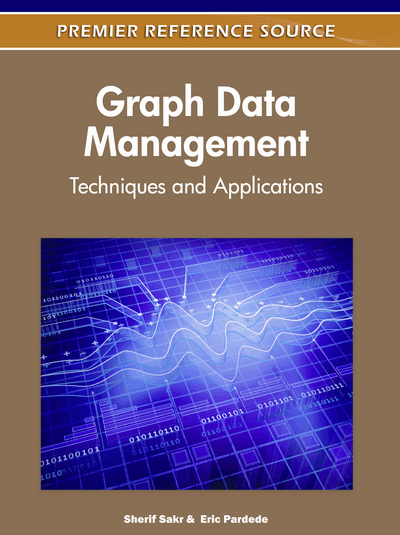# Matrix Decomposition-Based Dimensionality Reduction on Graph Data

Hiroto Saigo (Kyushu Institute of Technology, Japan) and Koji Tsuda (National Institute of Advanced Industrial Science and Technology (AIST), Japan)
DOI: 10.4018/978-1-61350-053-8.ch011
Available
\$37.50
No Current Special Offers

## Abstract

Graph is a mathematical framework that allows us to represent and manage many real-world data such as relational data, multimedia data and biomedical data. When each data point is represented as a graph and we are given a number of graphs, a task is to extract a few common patterns that capture the property of each population. A frequent graph mining algorithm such as AGM, gSpan and Gaston can enumerate all the frequent patterns in graph data, however, the number of patterns grows exponentially, therefore it is essential to output only discriminative patterns. There are many existing researches on this topic, but this chapter focus on the use of matrix decomposition techniques, and explains the two general cases where either i) no target label is available, or ii) target label is available for each data point. The reuslting method is a branch and bound pattern mining algorithm with efficient pruning condition, and we evaluate its effectiveness on cheminformatics data.
Chapter Preview
Top

## Introduction

Graph is a powerful mathematical framework which enables us to represent and manage various real-world objects in a natural way. The examples include XML, social networks and biological networks. In this chapter, we focus on applications in chemoinformatics where a chemical compound is represented as a labeled graph in which atoms and edges have corresponding labels such as H, C, N and single, double, aromatic bond, respectively. Given a large number of such graphs, a recent approach to extract common features is through the use of frequently appearing subgraphs. Even though it involves subgraph isomorphism problem, which is NP-hard, after the pioneering work AGM (Inokuchi, 2005), several fast frequent graph miners such as Gaston (Nijssen & Kok, 2004), gSpan gSpan (Yan & Han, 2002a) are proposed. A frequent subgraph mining algorithm enumerates all the subgraph patterns that appear more than m times in a graph database. The threshold m is called minimum support. As a next step after mining frequent patterns, we consider learning rules to classify graphs into positive class and negative class according to given labels. In order to achieve the best classification accuracy when classifying graph data, the minimum support threshold (the number of times a subgraph appears in graph data) has to be set to a small value (Wale & Karypis, 2006; Kazius, Nijssen, Kok, Baeck, & Ijzerman, 2006; Helma, Cramer, Kramer, & Raedt, 2004). However, such a setting creates millions of patterns, leading to difficulty when storing patterns and using them in the subsequent learning step. To avoid creating large number of uninformative patterns, we make use of a tree-shaped search space for enumerating frequent patterns (Figure 4). As we go down the tree from the root node, we encounter many child nodes corresponding to supergraphs of the parent node. For an efficiency reason, we desire to traverse only nodes that are necessary for the subsequent learning step.

Figure 4.

Classification accuracy (left) and computational time (right) against maximum pattern size (maxpat) in the CPDB dataset. In the table on the right, “Mining Time” stands for computational time for pattern search, and “Numerical Time” stands for computational time for matrix and vector operations.

In this chapter, we assume that the occurrence of subgraphs mined so far are recorded in a design matrix X. Since the number of subgraphs can be very large, the size of the design matrix can be too large to store in memory. Thereby we employ a so called matrix-free method, which constructs only a part of the whole matrix in an iterative fashion. More precisely, we make advantage of Lanczos method for unsupervised learning, and partial least squares (PLS) regression for supervised learning. The connection between the two appears later in this chapter.

Although we only deal with an application to chemical informatics data in this chapter, the proposed approach is applicable to other data such as protein (Jin, Young, & Wang, 2009), RNA (Tsuda & Kudo, 2006), text (Kudo, Maeda, & Matsumoto, 2005), image (Nowozin, Tsuda, Uno, Kudo, & Bakir, 2007), video (Nowozin, Bakir, & Tsuda, 2007) and so forth.

Top

As a next step after mining frequent patterns, many researchers have worked on integrating frequent pattern mining and rule learning algorithm. They are roughly categorized into filter approach and wrapper approach (Kohavi & John, 1997).

A filter approach first enumerates all the frequent patterns, then perform learning step afterwards (PatClass (Cheng, Yan, Han, & Hsu, 2007)). A pro of this approach is that one can employ recent learning algorithms without further change. Fei and Huan considered the spatial distribution of patterns for classifying graph data (Pattern SFS (Fei & Huan, 2009), LPGB (Fei & Huan, 2010)). A major con of filter approach is that the number of frequent pattern can become too large to perform the subsequent learning step because of a memory and storage issue.

## Complete Chapter List

Search this Book:
Reset